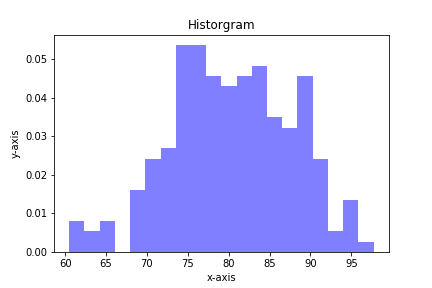## Plotting a Stress Strain Curve with Python and MatplotlibIn this post, we'll use data from a tensile test to build a stress strain curve with Python and Matplotlib.

A tensile test is a type of mechanical test performed by engineers in order to determine the mechanical properties of a material. Engineering metal alloys such as steel and aluminum …

## Plotting Bond Energy vs. Distance with Python and MatplotlibAtoms in solid materials like steel and aluminum are held together with chemical bonds. Atoms of solid materials are more stable when they are chemically bonded together, and it takes energy to separate atoms which are joined together with a chemical bond. The bonding energy associated with a chemical bond …

## Plotting a Gaussian normal curve with Python and MatplotlibIn the previous post, we calculated the area under the standard normal curve using Python and the erf() function from the math module in Python's Standard Library. In this post, we will construct a plot that illustrates the standard normal curve and the area we calculated. To build the Gaussian …

## Quiver plots using Python, matplotlib and Jupyter notebooksA quiver plot is a type of 2D plot that shows vector lines as arrows. Quiver plots are useful in electrical engineering to visualize electrical potential and valuable in mechanical engineering to show stress gradients. In this post, we will build three quiver plots using Python, matplotlib, numpy, and Jupyter notebooks.

## Plotting Histograms with matplotlib and PythonHistograms are a useful type of statistics plot for engineers. A histogram is a type of bar plot that shows the frequency or number of values compared to a set of value ranges. Histogram plots can be created with Python and the plotting package matplotlib. The plt.hist() function creates …